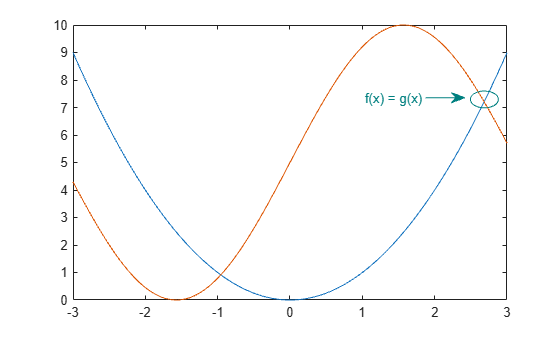Annotations are extra information added to a chart to help identify important information. This example first explains the different types of annotations, and then shows you how to add circles and text arrows to a chart.

### Types of Annotations

Use the `annotation` function to add annotations to a chart. The first input to the function specifies the type of annotation you want to create.

• If you specify the type as `'line'`, `'arrow'`, `'doublearrow'`, or `'textarrow'`, then the second input is the starting and ending x positions of the annotation. The third input is the starting and ending y positions of the annotation. For example, `annotation('line',[x_begin x_end],[y_begin y_end])`.

• If you specify the type as `'rectangle'`, `'ellipse'`, or `'textbox'`, then the second argument is the location and size. For example, `annotation('rectangle',[x y w h])`.

Annotations use normalized figure units and can span multiple axes in a figure.

### Create Simple Plot

Define and plot functions `f(x)` and `g(x)`.

```x = -3.0:0.01:3.0; f = x.^2; g = 5*sin(x) + 5; figure plot(x,f) hold on plot(x,g) hold off```### Circle Annotations

Add a circle to the chart to highlight where `f(x)` and `g(x)` are equal. To create a circle, use the `'ellipse'` option for the annotation type.

Customize the circle by setting properties of the underlying object. Return the `Ellipse` object as an output argument from the `annotation` function. Then, access properties of the object using dot notation. For example, set the `Color` property.

`elps = annotation('ellipse',[.84 .68 .05 .05])`
```elps = Ellipse with properties: Color: [0 0 0] FaceColor: 'none' LineStyle: '-' LineWidth: 0.5000 Position: [0.8400 0.6800 0.0500 0.0500] Units: 'normalized' Show all properties ```
`elps.Color = [0 0.5 0.5];`### Text Arrow Annotations

Add a text arrow to the chart using the `'textarrow'` option for the annotation type.

You can customize the text arrow by setting properties of the underlying object. Return the `TextArrow` object as an output argument from the `annotation` function. Then, access properties of the object using dot notation. For example, set the `String` property to the desired text and the `Color` property to a color value.

`ta = annotation('textarrow', [0.76 0.83], [0.71 0.71])`
```ta = TextArrow with properties: String: {''} FontName: 'Helvetica' FontSize: 10 Color: [0 0 0] TextColor: [0 0 0] LineStyle: '-' LineWidth: 0.5000 HeadStyle: 'vback2' Position: [0.7600 0.7100 0.0700 0] Units: 'normalized' X: [0.7600 0.8300] Y: [0.7100 0.7100] Show all properties ```
```ta.String = 'f(x) = g(x) '; ta.Color = [0 0.5 0.5]; ```